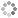# 7. Genesis of the Golden Ratio

by Dr. Bruce McLaughlin

This article presents evidence suggesting that the Golden Ratio is encrypted in the first three characters of the first verse of Genesis.

Introduction

A Judeo-Christian tradition is that God arranged the 304,805 character string of concatenated words in the Torah to reveal not only a spiritual message but also to encrypt fundamental information about the beginning of the universe and its development over time including the entirety of physics, chemistry, biology and human history… a message within a message.

In Genesis of the Reciprocal Fine Structure Constant by B. McLaughlin, several critical dimensionless numbers of physics/mathematics are predicted from the first 12 characters of the first verse of Genesis.  This article takes a closer look at the origin of the Golden Ratio which is not only one of the fundamental constants of mathematics but also of physics, chemistry and biology; it is revealed throughout the structure of the universe and within the complexity of life itself.  The Golden Ratio and associated Fibonacci sequence, Golden Angle and Golden Spiral are observed in: flower pedals, flower seed heads, pinecones, fruits and vegetables, tree branches, nautilus shells, inner ear cochlea, spiral galaxies, hurricanes, faces, fingers, animal bodies, reproductive dynamics, animal flight patterns, internal organ shape, DNA molecules and much more.

Is it possible that the Golden Ratio is encrypted in the first three characters of the first verse of Genesis?  What a perfect place to begin the encryption of a user manual revealing the physics and mathematics of this new creation from nothing (bara).

Background

The mathematical origin of the Golden Ratio generally begins with

(a + b)/a = a/b

which is equivalent to

(a/b) + 1 = (a/b)2

This means the Golden Ratio is a solution of x2 – x – 1 = 0.  The two solutions are

Φ = [1 + 51/2]/2

ψ = [1 - 51/2]/2

where Φ is the Golden Ratio and ψ is the Conjugate Golden Ratio.  They are related by

ψ = 1 - Φ = - (1/Φ)

The Golden Ratio also defines π by virtue of

π = (5)cos-1(Φ/2)

But what if the Golden Ratio is encrypted in the first three characters of the first verse of Genesis by a completely different mathematical path?

Analysis

The first three characters from the first verse of Genesis are

[Aleph][Resh][Bet]

reading from right to left.  Now invert the sequence and represent each character by a base-3 triplet (column vector) according to a rule that starts with Aleph as (000) and ends with Tsadey Final as (222).  These three base-3 triplets create the following 3 by 3 matrix having the elements 0, 1 and 2.

0 2 0

0 0 0

1 1 0

This matrix times its transpose yields

4 0 2

0 0 0

2 0 2

The three eigenvalues of this matrix product are:

3 + 51/2 = 2(1 + Φ) = 2Φ2

3 - 51/2 = 2(1 + ψ) = 2ψ2

0

The three eigenvectors of this matrix product can be written as multiples of:

(Φ,0,1)

(ψ,0,1)

(0,1,0)

Clearly, the Golden Ratio and its conjugate are encrypted in eigenvalues generated from the first three characters of the first verse of Genesis.  The eigenvectors also contain, as components, multiples of the Golden Ratio and its conjugate.

Conclusions

The hidden presence of the Golden Ratio in the first three characters, of the 304,805 character string of concatenated words in the Torah, might be a message that the Golden Ratio and associated Fibonacci sequence, Golden Angle, Golden Spiral and, of course, π are major mathematical, physical, chemical and biological building blocks of creation.  Or, it might be just one more curious coincidence!

If the encryption of the Golden Ratio in the first three characters of the first verse of Genesis is simply an interesting concurrence of circumstances without causal connection, then it seems unlikely that the Golden Ratio would be encrypted elsewhere in the first verse of Genesis.  This is particularly true if we adhere to the idea that the Genesis encryption, like the Genetic Code, is a non-overlapping triplet code.  The first verse of Genesis has 28 characters comprising only nine, non-overlapping groups of three characters plus Tsadey Final.  But the Golden Ratio is encrypted in at least three of the nine groups.  The third group of three characters is identical to the first group and the Appendix below shows that the Golden Ratio is also encrypted in the fifth group of three characters.

Appendix

The fifth three characters from the first verse of Genesis are

[Aleph][Mem Final][Yod]

reading from right to left.  Now invert the sequence and represent each character by a base-3 triplet (column vector) according to a rule that starts with Aleph as (000) and ends with Tsadey Final as (222).  These three base-3 triplets create the following 3 by 3 matrix having the elements 0, 1 and 2.

1 2 0

0 1 0

0 2 0

This matrix times its transpose yields

5 2 4

2 1 2

4 2 4

The three eigenvalues of this matrix product are

5 + 2(51/2) = 1 + 2(1 + 2Φ) = 1 + 2Φ3

5 - 2(51/2) = 1 + 2(1 + 2Ψ) = 1 + 2Ψ3

0

Therefore, the Golden Ratio is encrypted in the fifth three characters of the first verse of Genesis as well as in the first three and third three characters.